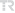## Exact and nonstandard finite difference schemes for the Burgers equation B(2,2)

TURKISH JOURNAL OF MATHEMATICS, vol.45, no.2, pp.647-660, 2021 (SCI-Expanded)• Publication Type: Article / Article
• Volume: 45 Issue: 2
• Publication Date: 2021
• Doi Number: 10.3906/mat-2009-51
• Journal Name: TURKISH JOURNAL OF MATHEMATICS
• Journal Indexes: Science Citation Index Expanded (SCI-EXPANDED), Scopus, Academic Search Premier, MathSciNet, zbMATH, TR DİZİN (ULAKBİM)
• Page Numbers: pp.647-660
• Hacettepe University Affiliated: Yes

#### Abstract

In this paper, we consider the Burgers equation B(2, 2) . Exact and nonstandard finite difference schemes (NSFD) for the Burgers equation B(2, 2) are designed. First, two exact finite difference schemes for the Burgers equation B(2, 2) are proposed using traveling wave solution. Then, two NSFD schemes are represented for this equation. These two NSFD schemes are compared with a standard finite difference (SFD) scheme. Numerical results show that the NSFD schemes are accurate and efficient in the numerical simulation of the kink-wave solution of the B(2, 2) equation. We see that although the SFD scheme yields numerical instability for large step sizes, NSFD schemes provide reliable results for long time integration. Local truncation errors show that the NSFD schemes are consistent with the B(2, 2) equation.